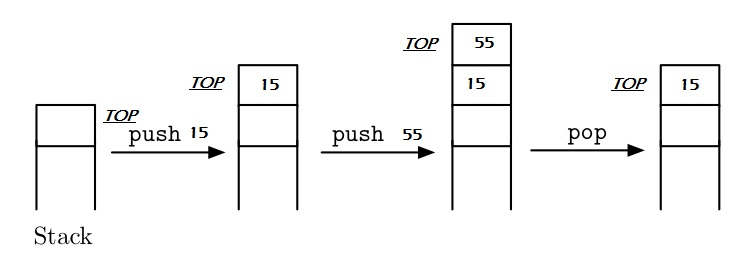# Stack Tutorial using C, C++ programs

## What is Stack?

• It is type of linear data structure.
• It follows LIFO (Last In First Out) property.
• It has only one pointer TOP that points the last or top most element of Stack.
• Insertion and Deletion in stack can only be done from top only.
• Insertion in stack is also known as a PUSH operation.
• Deletion from stack is also known as POP operation in stack.

### Stack Implementation

• Stack implementation using array.
• Stack implementation using linked list.

### Applications of Stack

• Conversion ofpolish notations
There are three types of notations:
> Infix notation - Operator is between the operands : x + y
> Prefix notation - Operator is before the operands : + xy
> Postfix notation - Operator is after the operands : xy +
• To reverse a string
A string can be reversed by using stack. The characters of string pushed on to the stack till the end of the string. The characters are popped and displays. Since the end character of string is pushed at the last, it will be printed first.
• When function (sub-program) is called
When a function is called, the function is called last will be completed first. It is the property of stack. There is a memory area, specially reserved for this stack.

### Declaration of Stack

```    /*stack declaration*/
typedef struct
{
int   top;
int   items[MAX];
}Stack;
```
```    #define MAX 5

//Declaration of Stack
class Stack
{
private:
int top;
int ele[MAX];
public:
Stack();
int 	isFull();
int 	isEmpty();
void    push(int item);
int     pop(int *item);
};
```

#### Stack representation#### Functions and Algorithms

• Initialization of stack.
• Insertion into stack ( push operation).
• Deletion from stack (pop operation).
• Check fullness.
• Check emptiness.

#### Algorithms

In stack related algorithms TOP initially point 0, index of elements in stack is start from 1, and index of last element is MAX.

```    INIT_STACK (STACK, TOP)

Algorithm to initialize a stack using array.
TOP points to the top-most element of stack.

1) TOP: = 0;
2) Exit
```

Push operation is used to insert an element into stack.

```    PUSH_STACK(STACK,TOP,MAX,ITEM)

Algorithm to push an item into stack.

1) IF TOP = MAX   then
Print “Stack is full”;
Exit;
2) Otherwise
TOP: = TOP + 1;        /*increment TOP*/
STACK (TOP):= ITEM;
3) End of IF
4) Exit
```

Pop operation is used to remove an item from stack, first get the element and then decrease TOP pointer.

```    POP_STACK(STACK,TOP,ITEM)

Algorithm to pop an element from stack.

1) IF TOP = 0 then
Print “Stack is empty”;
Exit;
2) Otherwise
ITEM: =STACK (TOP);
TOP:=TOP – 1;
3) End of IF
4) Exit
```
```    IS_FULL(STACK,TOP,MAX,STATUS)

Algorithm to check stack is full or not.
STATUS contains the result status.

1) IF TOP = MAX then
STATUS:=true;
2) Otherwise
STATUS:=false;
3)  End of IF
4)  Exit
```
```    IS_EMPTY(STACK,TOP,MAX,STATUS)

Algorithm to check stack is empty or not.
STATUS contains the result status.

1) IF TOP = 0 then
STATUS:=true;
2) Otherwise
STATUS:=false;
3)  End of IF
4)  Exit
```

#### Complete program to implement stack using above functions & algorithms

```    #include<stdio.h>
#define MAX 3

typedef struct
{
int TOP;
int ele[MAX];

}Stack;

void init(Stack *s)
{
s->TOP = -1;
}

int isFull(Stack *s)
{
if(s->TOP == MAX-1)
return 0;
return -1;
}

int isEmpty(Stack *s)
{
if(s->TOP == -1)
return 0;
return -1;
}

void push(Stack *s, int item)
{
if( !isFull(s) )
{
printf("\nStack is full");
return;
}
s->TOP = s->TOP + 1;
s->ele[s->TOP] = item;
}

int pop(Stack *s, int *item)
{
if(!isEmpty(s))
{
printf("\nStack is empty");
return -1;
}
*item = s->ele[s->TOP];
s->TOP = s->TOP - 1;

return 0;

}

int main()
{
Stack s;
int item;

clrscr();

init(&s);

push(&s,10);
push(&s,20);
push(&s,30);

pop(&s,&item);
printf("\nPoped Item : %d",item);

pop(&s,&item);
printf("\nPoped Item : %d",item);

pop(&s,&item);
printf("\nPoped Item : %d",item);
getch();

return 0;
}
```
```    Inserted item : 10
Inserted item : 20
Inserted item : 30
Inserted item : 40
Inserted item : 50
Stack Overflow
Deleted item : 50
Deleted item : 40
Deleted item : 30
Deleted item : 20
Deleted item : 10
Stack Underflow
```
```    #include <iostream>
using namespace std;
#define MAX 5

//Declaration of Stack
class Stack
{
private:
int top;
int ele[MAX];
public:
Stack();
int 	isFull();
int 	isEmpty();
void    push(int item);
int     pop(int *item);
};

//Initialization of stack
Stack:: Stack()
{
top = -1;
}

//Check stack is full or not
int Stack:: isFull()
{
int full = 0;

if( top == MAX-1 )
full = 1;

return full;
}

//Check stack is empty or not
int Stack:: isEmpty()
{
int empty = 0;

if( top == -1 )
empty = 1;

return empty;
}

//Insert item into stack
void Stack:: push( int item )
{
if( isFull() )
{
cout<<"\nStack Overflow";
return;
}

top++;
ele[top] = item;

cout<<"\nInserted item : "<< item;
}

//Delete item from stack
int Stack:: pop( int *item )
{
if( isEmpty() )
{
cout<<"\nStack Underflow";
return -1;
}

*item = ele[top--];
return 0;
}

int main()
{
int item = 0;

Stack s = Stack();

s.push( 10 );
s.push( 20 );
s.push( 30 );
s.push( 40 );
s.push( 50 );
s.push( 60 );

if( s.pop(&item) == 0 )
cout<<"\nDeleted item : "<< item;
if( s.pop(&item) == 0 )
cout<<"\nDeleted item : "<< item;
if( s.pop(&item) == 0 )
cout<<"\nDeleted item : "<< item;
if( s.pop(&item) == 0 )
cout<<"\nDeleted item : "<< item;
if( s.pop(&item) == 0 )
cout<<"\nDeleted item : "<< item;
if( s.pop(&item) == 0 )
cout<<"\nDeleted item : "<< item;

cout<< endl;

return 0;
}
```
```    Inserted item : 10
Inserted item : 20
Inserted item : 30
Inserted item : 40
Inserted item : 50
Stack Overflow
Deleted item : 50
Deleted item : 40
Deleted item : 30
Deleted item : 20
Deleted item : 10
Stack Underflow
```